# 当代程序员必备技能（算法）之：递归详解Java架构师迁哥

# 前言

• 什么是递归？

• 递归的特点

• 递归与栈的关系

• 递归应用场景

• 递归解题思路

• leetcode案例分析

• 递归可能存在的问题以及解决方案

# 什么是递归？

public int sum(int n) {    if (n <= 1) {        return 1;    }     return sum(n - 1) + n; }﻿

﻿

# 递归的特点

• 自身调用：原问题可以分解为子问题，子问题和原问题的求解方法是一致的，即都是调用自身的同一个函数。

• 终止条件：递归必须有一个终止的条件，即不能无限循环地调用本身。

﻿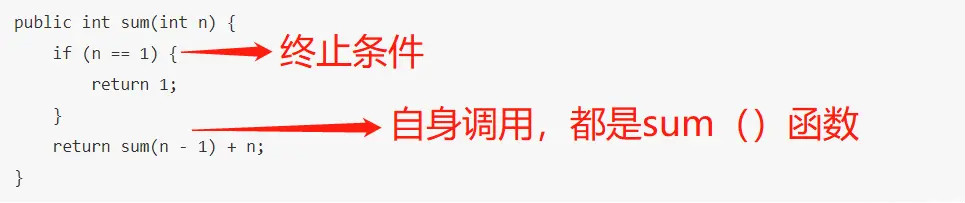﻿

# 递归与栈的关系

﻿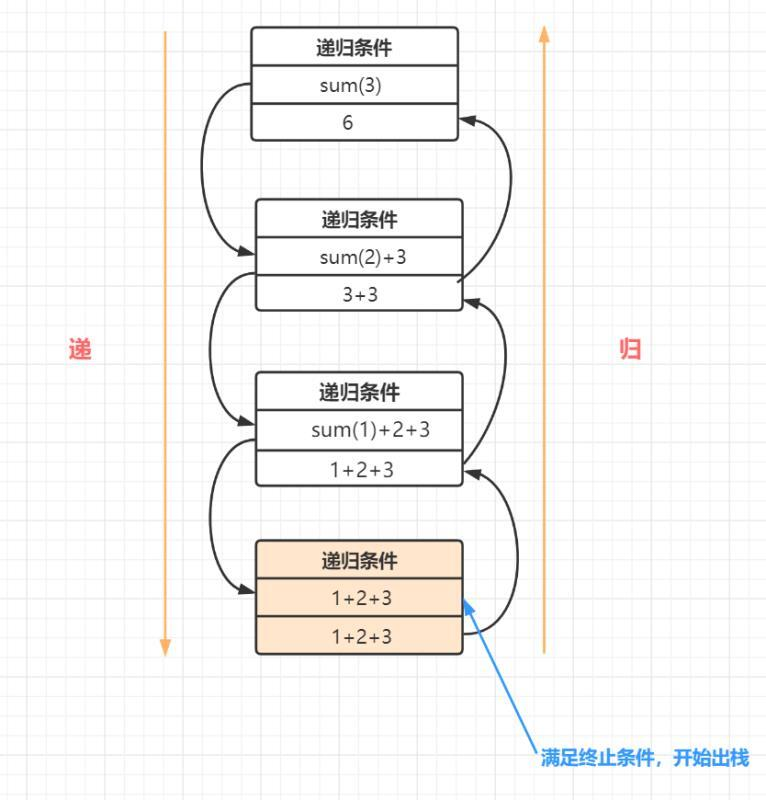﻿

﻿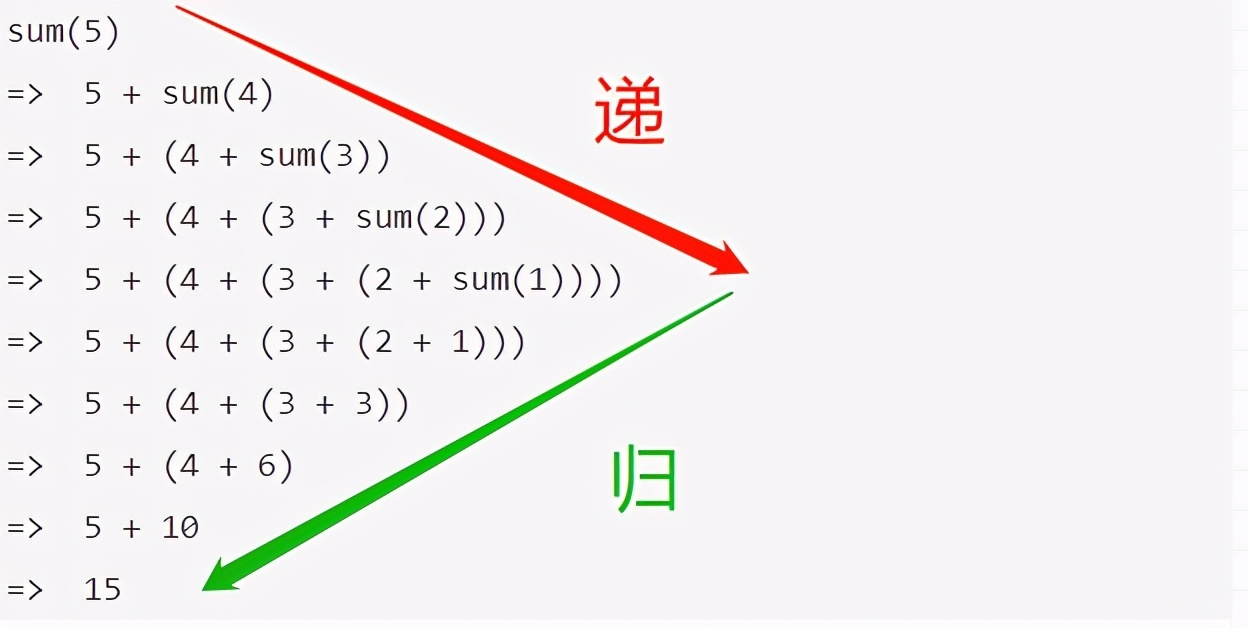﻿

• 计算sum（5）时，先sum（5）入栈，然后原问题sum（5）拆分为子问题sum（4），再入栈，直到终止条件sum（n=1）=1，就开始出栈。

• sum（1）出栈后，sum（2）开始出栈，接着sum（3）。

• 最后呢,sum（1）就是后进先出，sum（5）是先进后出，因此递归过程可以理解为栈出入过程啦~

# 递归的经典应用场景

• 阶乘问题

• 二叉树深度

• 汉诺塔问题

• 斐波那契数列

• 快速排序、归并排序（分治算法也使用递归实现）

• 遍历文件，解析xml文件

# 递归解题思路

• 第一步，定义函数功能

• 第二步，寻找递归终止条件

• 第二步，递推函数的等价关系式

# 1.定义函数功能

//n的阶乘（n为大于0的自然数）int factorial (int n){﻿}﻿

﻿

# 2.寻找递归终止条件

//n的阶乘（n为大于0的自然数）int factorial (int n){    if(n==1){      return 1;    }}﻿

﻿

# 3.递推函数的等价关系式

int factorial (int n){    if(n==1){      return 1;    }    return n * factorial(n-1);}﻿

﻿

「注意啦」，不是所有递推函数的等价关系都像阶乘这么简单，一下子就能推导出来。需要我们多接触，多积累，多思考，多练习递归题目滴~

# leetcode案例分析

「题目：」 翻转一棵二叉树。

     4   /   \  2     7 / \   / \1   3 6   9﻿

﻿

     4   /   \  7     2 / \   / \9   6 3   1﻿

﻿

「1. 定义函数功能」

//翻转一颗二叉树public TreeNode invertTree(TreeNode root) {}﻿/** * Definition for a binary tree node. * public class TreeNode { *     int val; *     TreeNode left; *     TreeNode right; *     TreeNode(int x) { val = x; } * } */﻿

﻿

「2.寻找递归终止条件」

//翻转一颗二叉树public TreeNode invertTree(TreeNode root) {    if(root==null || (root.left ==null && root.right ==null)){       return root;    }}﻿

﻿

「3. 递推函数的等价关系式」

﻿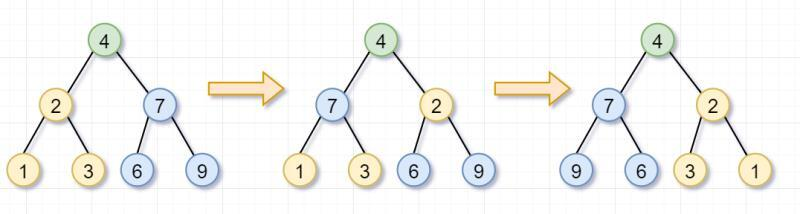﻿

﻿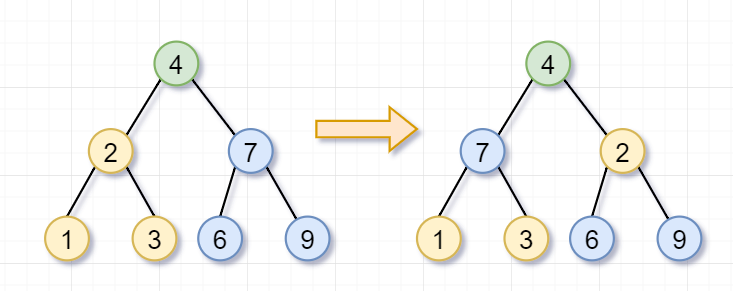﻿

﻿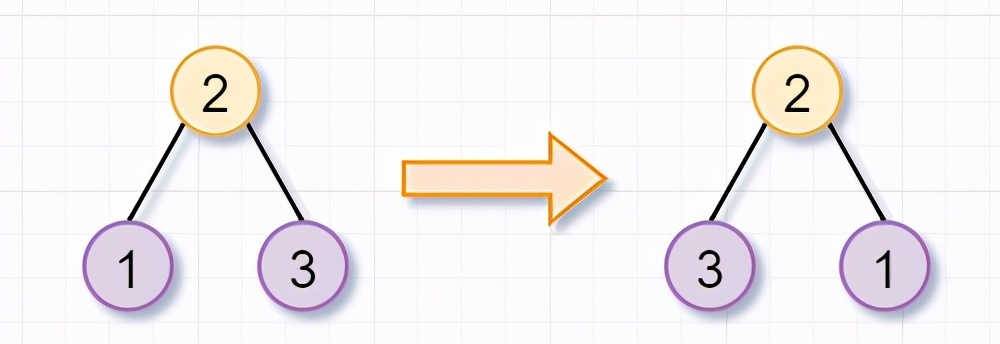﻿

﻿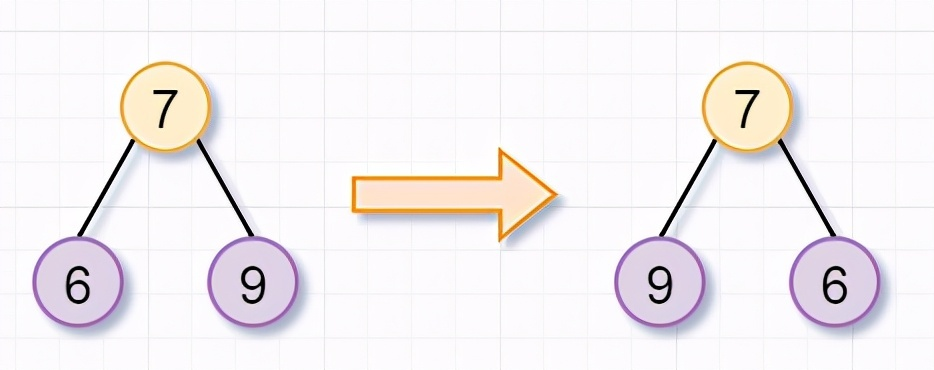﻿

﻿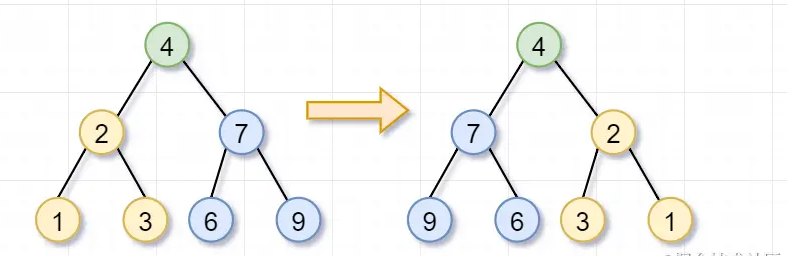﻿

invertTree（root）= invertTree（root.left） + invertTree（root.right）;﻿

﻿

//翻转一颗二叉树public TreeNode invertTree(TreeNode root) {    if(root==null || (root.left ==null && root.right ==null){       return root;    }    //翻转左子树    TreeNode left = invertTree(root.left);    //翻转右子树    TreeNode right= invertTree(root.right);}﻿

﻿

 root.left = right; root.right = left;﻿

﻿

class Solution {    public TreeNode invertTree(TreeNode root) {         if(root==null || (root.left ==null && root.right ==null)){           return root;         }         //翻转左子树         TreeNode left = invertTree(root.left);         //翻转右子树         TreeNode right= invertTree(root.right);         //左右子树交换位置~         root.left = right;         root.right = left;         return root;    }}﻿

﻿

﻿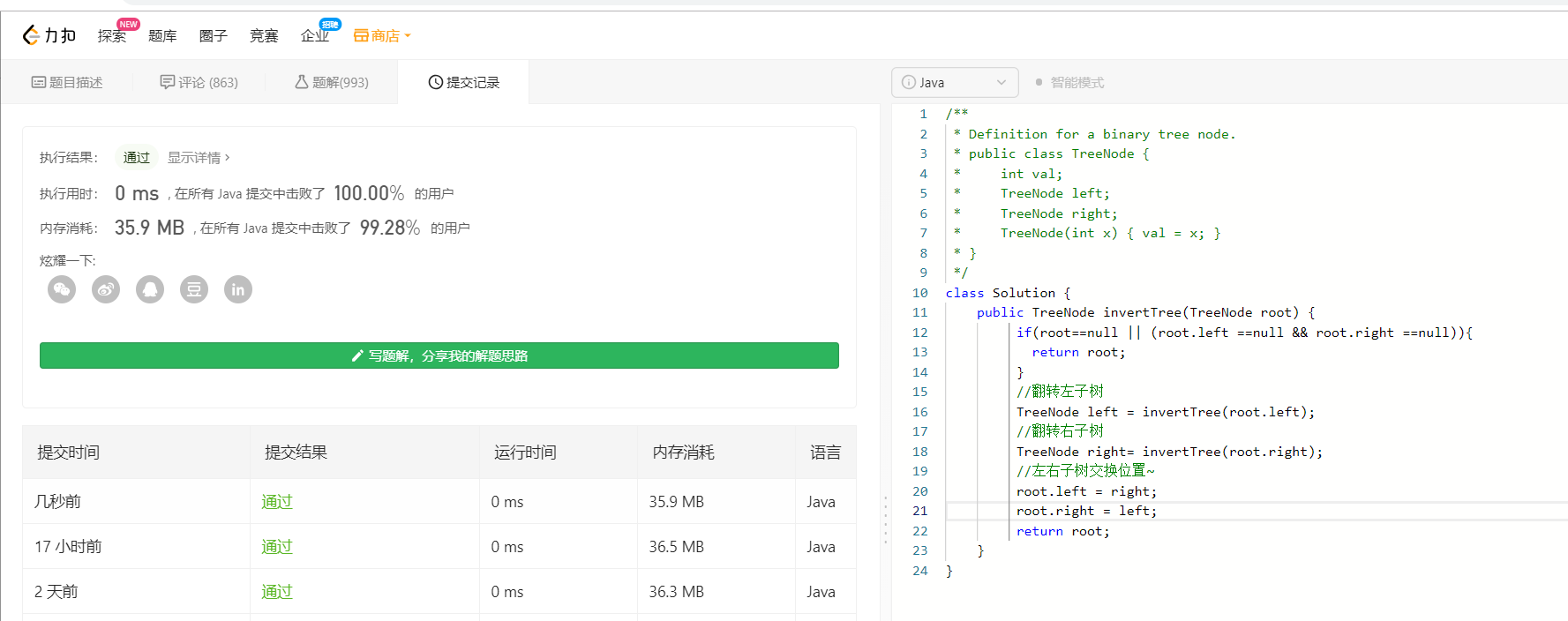﻿

# 递归存在的问题

• 递归调用层级太多，导致栈溢出问题

• 递归重复计算，导致效率低下

# 栈溢出问题

• 每一次函数调用在内存栈中分配空间，而每个进程的栈容量是有限的。

• 当递归调用的层级太多时，就会超出栈的容量，从而导致调用栈溢出。

• 其实，我们在前面小节也讨论了，递归过程类似于出栈入栈，如果递归次数过多，栈的深度就需要越深，最后栈容量真的不够咯

「代码例子如下：」

/** * 递归栈溢出测试 */public class RecursionTest {﻿    public static void main(String[] args) {        sum(50000);    }    private static int sum(int n) {        if (n <= 1) {            return 1;        }        return sum(n - 1) + n;    }}﻿

﻿

「运行结果:」

Exception in thread "main" java.lang.StackOverflowError at recursion.RecursionTest.sum(RecursionTest.java:13)﻿

﻿

# 重复计算，导致程序效率低下

class Solution {    public int numWays(int n) {    if (n == 0){       return 1;     }    if(n <= 2){        return n;    }    return numWays(n-1) + numWays(n-2);    }}﻿

﻿

﻿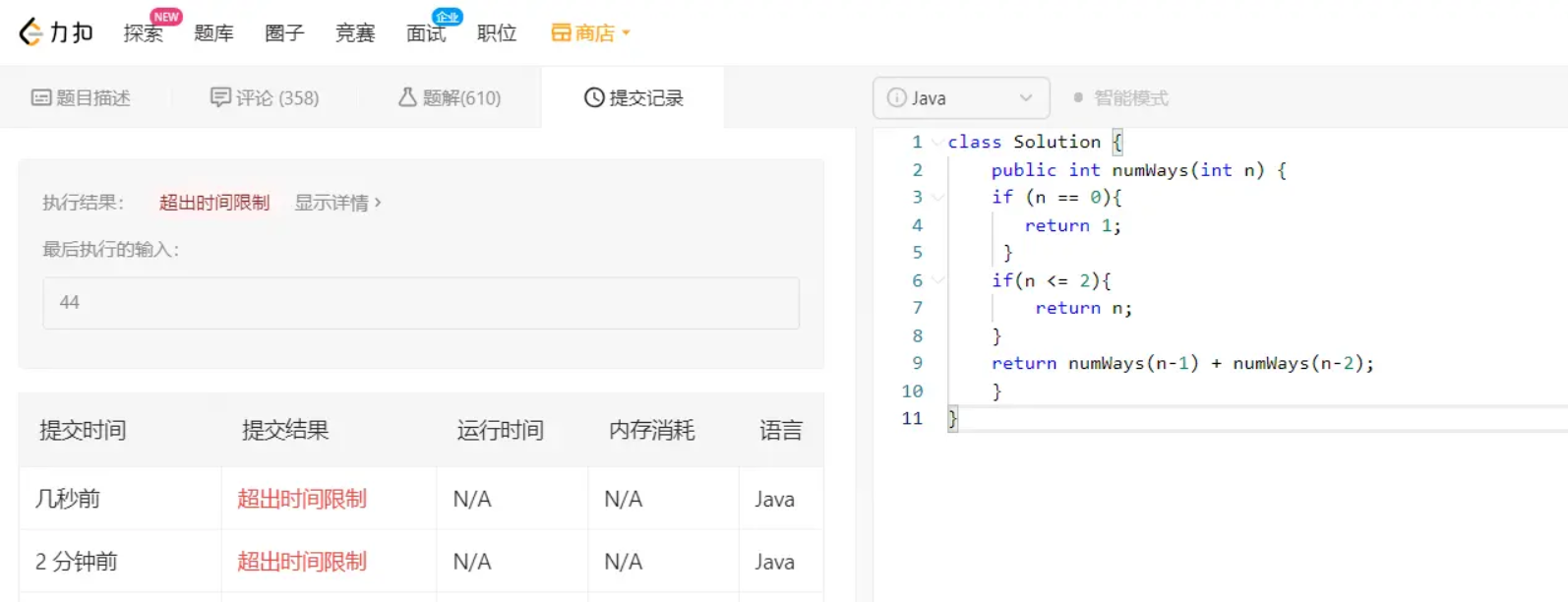﻿

﻿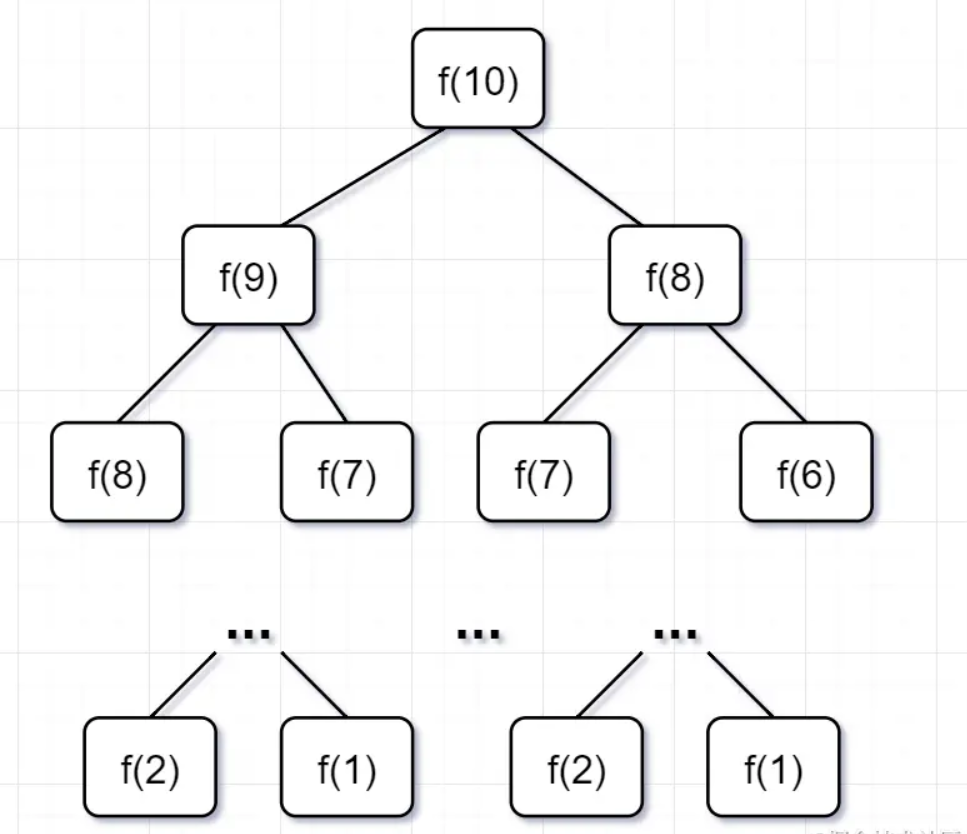﻿

• 要计算原问题 f(10)，就需要先计算出子问题 f(9) 和 f(8)

• 然后要计算 f(9)，又要先算出子问题 f(8) 和 f(7)，以此类推。

• 一直到 f(2) 和 f(1），递归树才终止。

• 一个子问题时间 = f（n-1）+f（n-2），也就是一个加法的操作，所以复杂度是 「O(1)」

• 问题个数 = 递归树节点的总数，递归树的总结点 = 2^n-1，所以是复杂度「O(2^n)」

「那么，怎么解决这个问题呢？」

「第一步」，f（10）= f(9) + f(8)，f(9) 和f（8）都需要计算出来，然后再加到备忘录中，如下：

﻿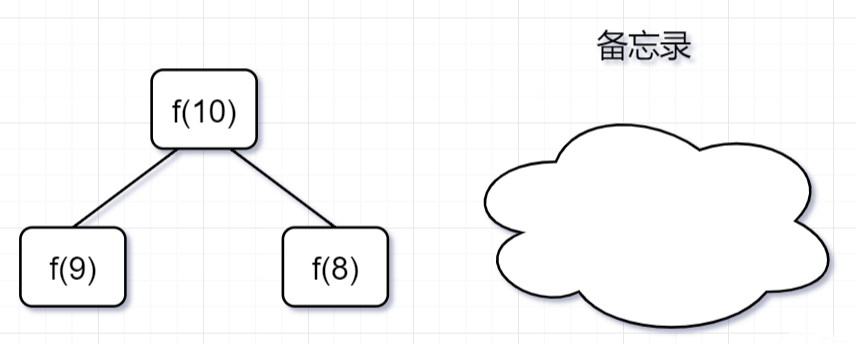﻿

「第二步，」 f(9) = f（8）+ f（7），f（8）= f（7）+ f(6), 因为 f(8) 已经在备忘录中啦，所以可以省掉，f(7),f（6）都需要计算出来，加到备忘录中~

﻿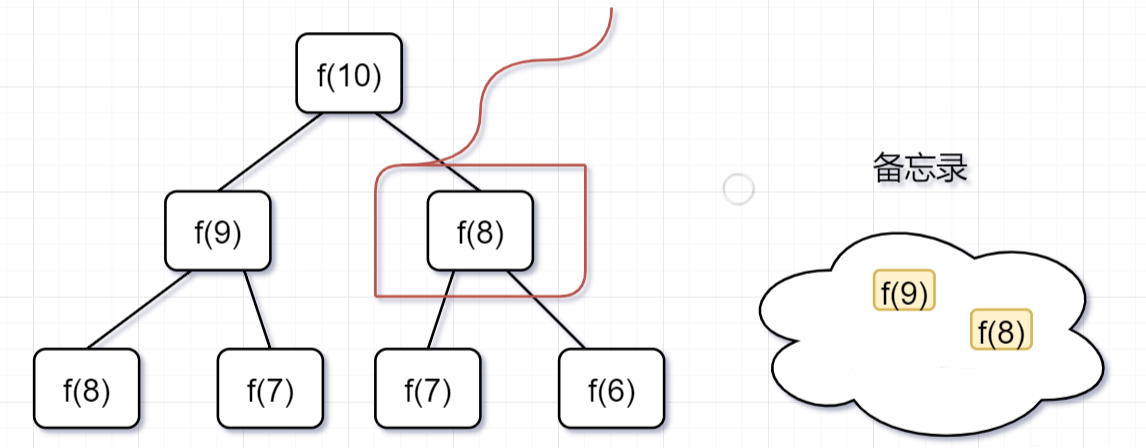﻿

「第三步，」 f(8) = f（7）+ f(6),发现f(8)，f(7),f（6）全部都在备忘录上了，所以都可以剪掉。

﻿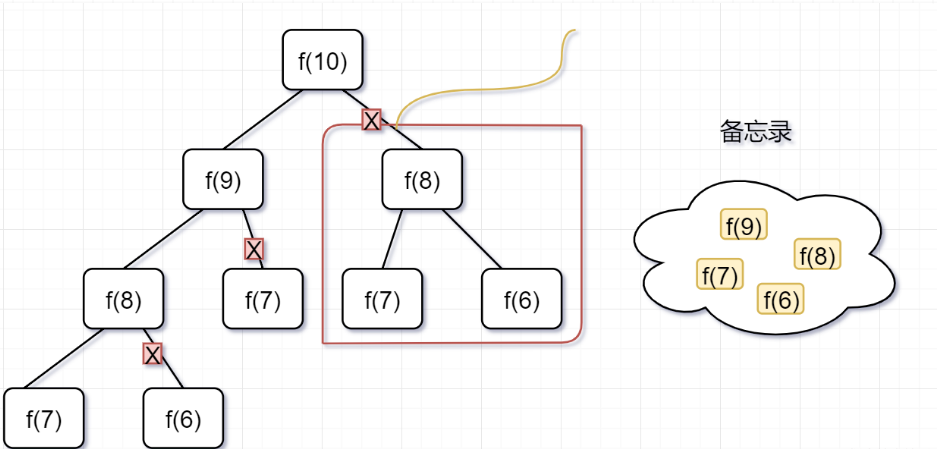﻿

﻿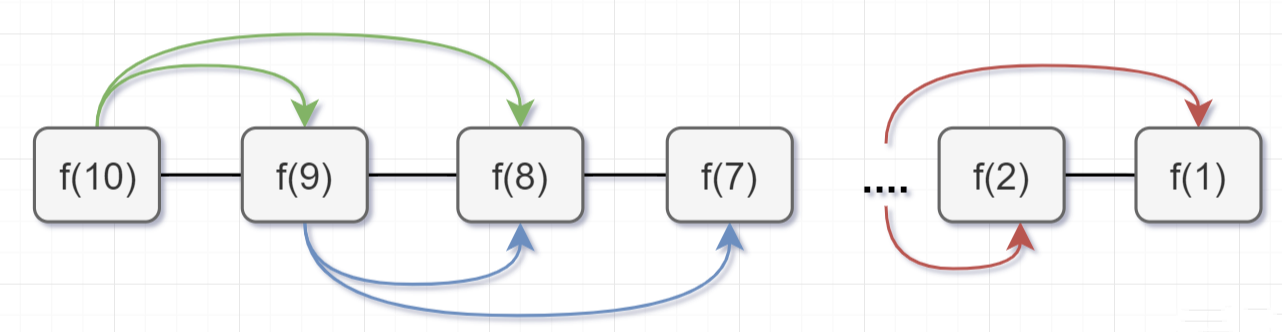﻿

public class Solution {    //使用哈希map，充当备忘录的作用    Map<Integer, Integer> tempMap = new HashMap();    public int numWays(int n) {        // n = 0 也算1种        if (n == 0) {            return 1;        }        if (n <= 2) {            return n;        }        //先判断有没计算过，即看看备忘录有没有        if (tempMap.containsKey(n)) {            //备忘录有，即计算过，直接返回            return tempMap.get(n);        } else {            // 备忘录没有，即没有计算过，执行递归计算,并且把结果保存到备忘录map中，对1000000007取余（这个是leetcode题目规定的）            tempMap.put(n, (numWays(n - 1) + numWays(n - 2)) % 1000000007);            return tempMap.get(n);        }    }}﻿

﻿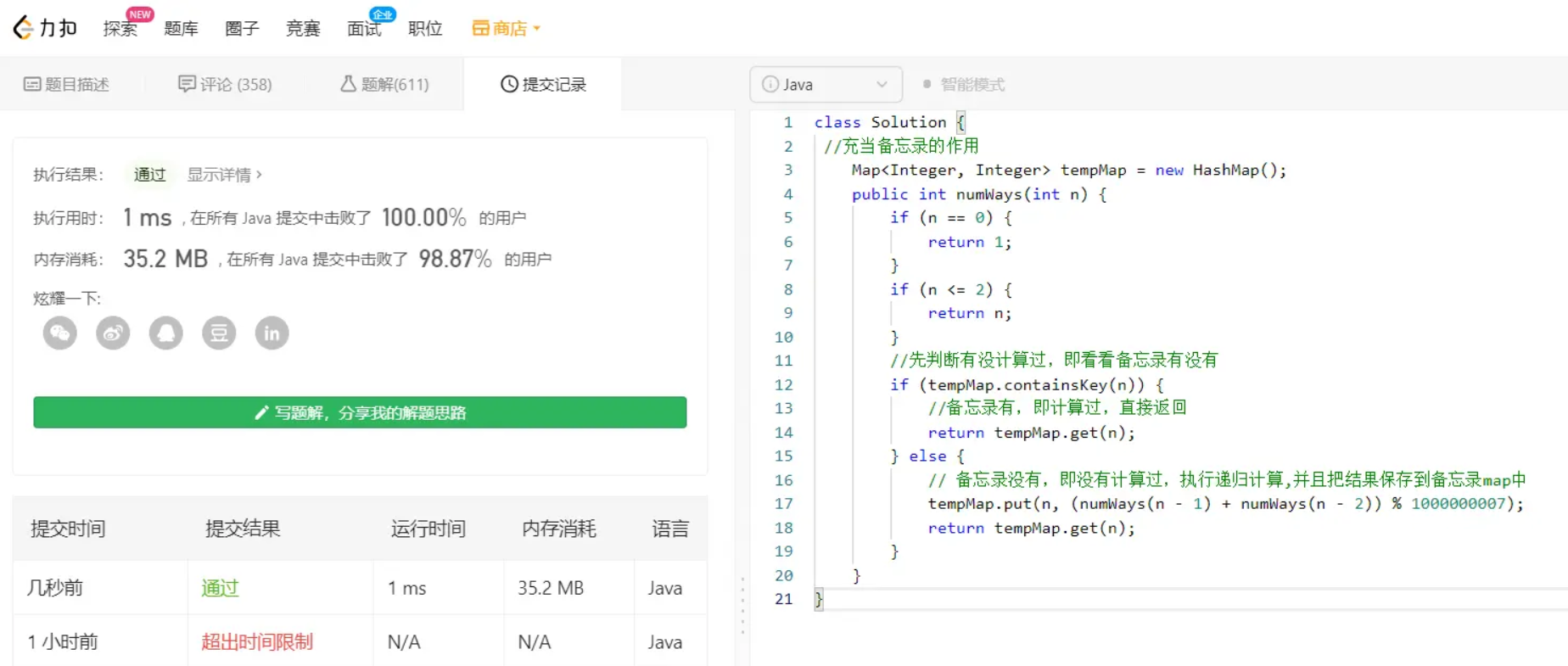﻿﻿

﻿

# 看完三件事❤️

========

﻿## 评论